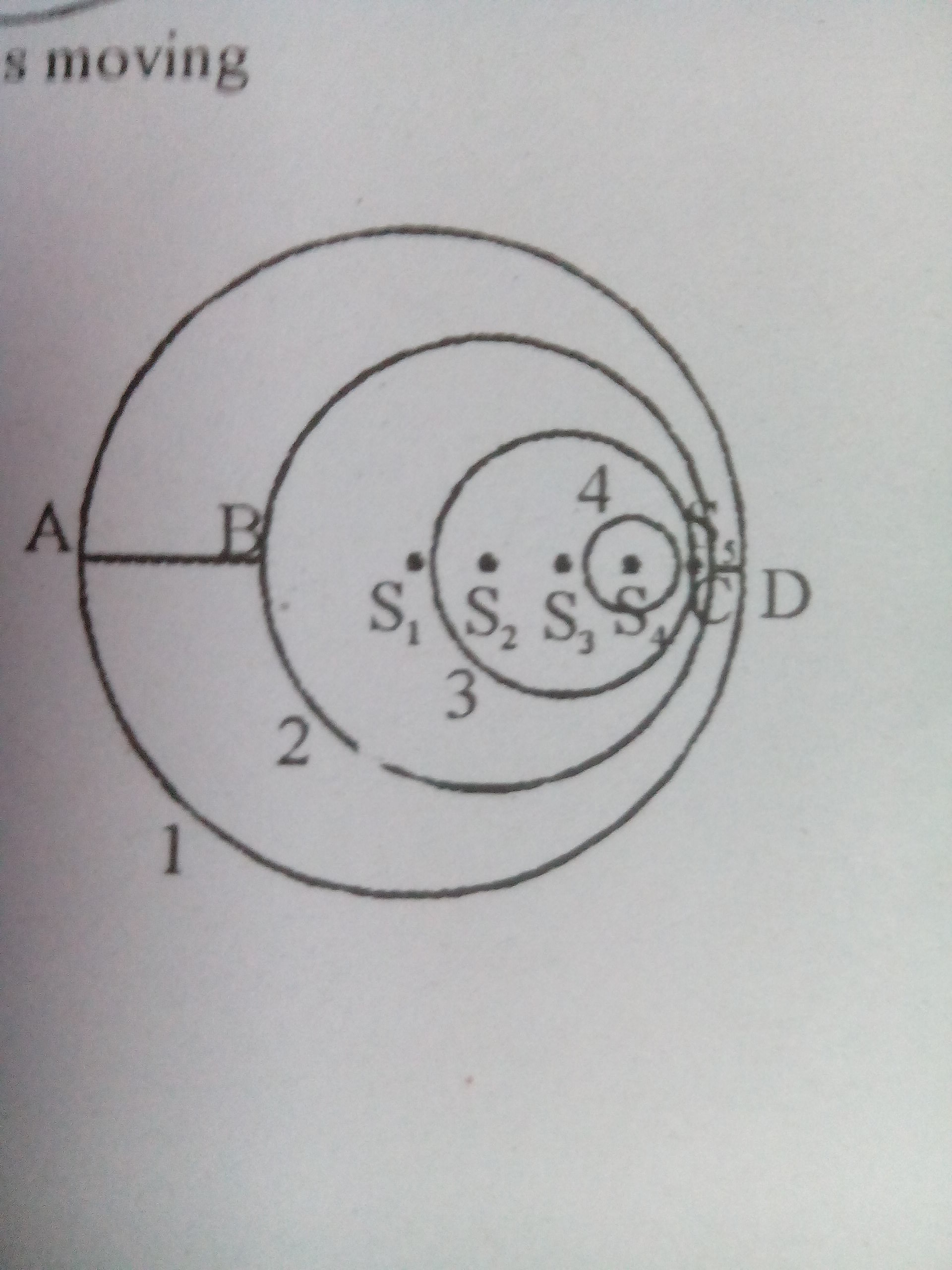# Help needed in thisLabels $$1,2,3,4,5$$ show wavefronts emitted successively at regular interval $$T=\frac{1}{f}$$ , $$f$$ is frequency, when the source is located at $$S_{1},S_{2},S_{3},S_{4},S_{5}$$ respectively. Each wavefront will have its centre at the position where the source was situated while emitting the wavefront. The radius of preceding wavefront will exceed the next one by an amount $$Vt$$. The wavefront for position $$S_{5}$$ is just being emitted and therefore it is a point

Consider the diagram above, $AB=\frac{4}{3}m$. Speed of sound and frequency are $330~m/s$ and $330~Hz$ respectively. The velocity of source is

In the above diagram value of $CD$ will beNote by Tanishq Varshney
6 years, 1 month ago

This discussion board is a place to discuss our Daily Challenges and the math and science related to those challenges. Explanations are more than just a solution — they should explain the steps and thinking strategies that you used to obtain the solution. Comments should further the discussion of math and science.

When posting on Brilliant:

• Use the emojis to react to an explanation, whether you're congratulating a job well done , or just really confused .
• Ask specific questions about the challenge or the steps in somebody's explanation. Well-posed questions can add a lot to the discussion, but posting "I don't understand!" doesn't help anyone.
• Try to contribute something new to the discussion, whether it is an extension, generalization or other idea related to the challenge.
• Stay on topic — we're all here to learn more about math and science, not to hear about your favorite get-rich-quick scheme or current world events.

MarkdownAppears as
*italics* or _italics_ italics
**bold** or __bold__ bold
- bulleted- list
• bulleted
• list
1. numbered2. list
1. numbered
2. list
Note: you must add a full line of space before and after lists for them to show up correctly
paragraph 1paragraph 2

paragraph 1

paragraph 2

[example link](https://brilliant.org)example link
> This is a quote
This is a quote
    # I indented these lines
# 4 spaces, and now they show
# up as a code block.

print "hello world"
# I indented these lines
# 4 spaces, and now they show
# up as a code block.

print "hello world"
MathAppears as
Remember to wrap math in $$ ... $$ or $ ... $ to ensure proper formatting.
2 \times 3 $2 \times 3$
2^{34} $2^{34}$
a_{i-1} $a_{i-1}$
\frac{2}{3} $\frac{2}{3}$
\sqrt{2} $\sqrt{2}$
\sum_{i=1}^3 $\sum_{i=1}^3$
\sin \theta $\sin \theta$
\boxed{123} $\boxed{123}$

## Comments

Sort by:

Top Newest

@Raghav Vaidyanathan @Ronak Agarwal @Nishant Rai , Answers are 110m/s and $CD=\frac{2}{3}m$

- 6 years, 1 month ago

Log in to reply

This is easy. The difference of the radii of the two different circles is given by $Wavelength$ since you see every wavefront is being released at time

1/f ( Distance=Speed Time , v1/f )

But the extra distance between A and B is coming because of the speed of the source

The extra distance is $\dfrac{{v}_{Source}}{f}$

With this difference you can calculate the speed.

Find CD is also easy since you can subtract the extra difference from the wavelength to get the answer.

- 6 years, 1 month ago

Log in to reply

can u elaborate the CD one, in the first one u mean to say $\frac{4}{3}-\frac{330}{330}=\frac{v_{source}}{f}$

- 6 years, 1 month ago

Log in to reply

ok i got its $CD-1=\frac{1}{3}$

- 6 years, 1 month ago

Log in to reply

×

Problem Loading...

Note Loading...

Set Loading...